# Vitali-Hahn-Saks theorem

(diff) ← Older revision | Latest revision (diff) | Newer revision → (diff)

Let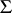be a-algebra (cf. also Borel field of sets). Letbe a non-negative set function and let, whereis a normed space. One says thatis absolutely continuous with respect to, denoted by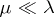, if for every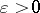there exists a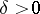such that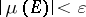whenever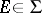and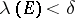(cf. also Absolute continuity). A sequenceis uniformly absolutely continuous with respect toif for everythere exists asuch thatwhenever,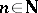and.

The Vitali–Hahn–Saks theorem [a7], [a2] says that for any sequence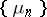of signed measures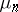which are absolutely continuous with respect to a measureand for whichexists for each, the following is true:

i) the limitis also absolutely continuous with respect to this measure, i.e.;

ii)is uniformly absolutely continuous with respect to. This theorem is closely related to integration theory [a8], [a3]. Namely, if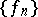is a sequence of functions from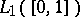, whereis the Lebesgue measure, and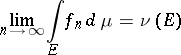exists for each measurable set, then the sequence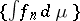is uniformly absolutely-continuous andis absolutely-continuous, [a3].

R.S. Phillips [a5] and C.E. Rickart [a6] have extended the Vitali–Hahn–Saks theorem to measures with values in a locally convex topological vector space (cf. also Locally convex space).

There are also generalizations to functions defined on orthomodular lattices and with more general properties ([a1], [a4]).

How to Cite This Entry:
Vitali-Hahn-Saks theorem. Encyclopedia of Mathematics. URL: http://encyclopediaofmath.org/index.php?title=Vitali-Hahn-Saks_theorem&oldid=13281
This article was adapted from an original article by E. Pap (originator), which appeared in Encyclopedia of Mathematics - ISBN 1402006098. See original article